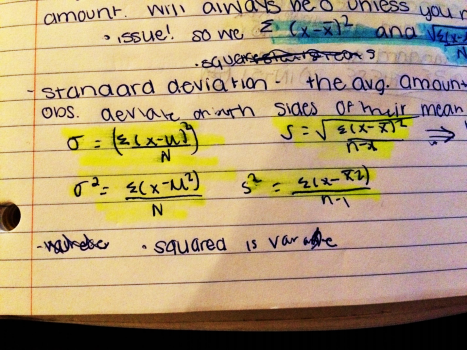×
Get Full Access to UA - GE 276 - Class Notes - Week 4
Get Full Access to UA - GE 276 - Class Notes - Week 4

×

UA / General Education / GE 276 / What are the 4 equations to know in standard deviation?

# What are the 4 equations to know in standard deviation? Description

##### Description: Class notes from week 4 of BNAD 276
2 Pages 87 Views 1 Unlocks
Reviews

BNAD 276 Week 4 Class Notes

## What are the 4 equations to know in standard deviation?∙ Deviation Score- average-number.

o 0 means average

o a negative number can be good or bad. It depends on the  situation it is in.

o Example: the average is 6’0”.

 6’2”= +2 above average

 5’8”= -4 below average

o (x-μ) x= your data and μ=average

∙ average deviation score- add up deviation scores and divide by  amount. It will always be 0 unless you did it wrong.

∙ To get a standard deviation you want to square the deviation, add it up  x−¿

¿

and square root it. (

## What is deviation score?¿2¿¿¿

Σ√¿

)

∙ Standard deviation- the average amount by which observations  deviate on either side of their mean  We also discuss several other topics like What are the three methodological approaches?

∙ 4 equations to know

o Notes:

o σ= sigma

o S and σ= standard deviation sample (s) and population (σ) o S^2 and σ^2= variance sample (s) and population (σ) x−¿

o Sum of squares=

¿

¿2¿Σ¿ ¿

## What is an average deviation score?and Σ√( x−x )2 n−1

o n-1= degree of separation∙ standard deviation squared= variance  ∙ n-1 is degrees of freedom Don't forget about the age old question of Why is evolution so important to biology?

o you lose a degree of freedom every time you guess (for every  parameter you estimate)

o it’s a mathematical correction because of estimating

∙ difference is standard deviation squared is variance. Variance square  rooted is standard deviation.

o Numerator= sum of squares.

∙ Example

X

Deviation

Standard deviation

1

-4

(-4)^2 = 16

2

-4

(-3)^2= 9

3

-2

(-2)^2= 4

4

-1

(-1)^2= 1

5

0

0^2= 0

6

1

1^2= 1

7

2

2^2= 4

8

3

3^2= 9

9

4

4^2= 16

Σ=45

60

Don't forget about the age old question of What is the conscious?

Deviation: square root of 60/9= 6.67 (this is the standard

deviation)

∙ 1 Standard deviation above and below mean is 68% of population ∙ 2 standard deviations above and below mean is 95% of population ∙ 3 standard deviations above and below mean is 99.7% of population Don't forget about the age old question of What is fragmentative analysis?
We also discuss several other topics like What inhibits the na/k pump?

∙ Z-Score

o 100% under curve

o 50% (0.5) Is above mean and 50% of below mean

o Why do we care about raw scores?

 Because they give us the ACTUAL number

o Proportions= percent of/ percentile  Don't forget about the age old question of What are the steps of the civil process?

o The raw scores and proportions do not correrlate naturally. But  they do both correrlate to Z scores so we change them to that to  compare!

∙ Z= x-μ/σ

Page ExpiredIt looks like your free minutes have expired! Lucky for you we have all the content you need, just sign up here